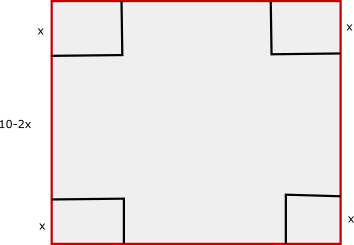Question 32

# A square piece of cardboard of sides ten inches is taken and four equal squares pieces are removed at the corners, such that the side of this square piece is also an integer value. The sides are then turned up to form an open box. Then the maximum volume such a box can have is

Solution

Let the side of the square which is cut be x.Volume of the cuboid so formed =$$(10-2x)^2*x$$

Put x = 1, 2, 3 and so on till 5

Maximum volume would be at x = 2

Volume of the cuboid so formed =$$(10-2*2)^2*2 = 72$$